# Types of Variables in Python

## Types of Variables in Python with Examples

In this article, I am going to discuss Types of Variables in Python with Examples. Please read our previous article where we discussed Function Arguments in Python with examples. At the end of this article, you will understand the following pointers in detail which are related to Types of Variables in Python.

1. Types of Variables in Python
2. Local Variables in Python
3. Global variables in Python
4. Global Keyword in Python
5. When can we choose a global keyword?
6. Globals() built-in function in python
##### Types of Variables in Python

The variables based on their scope can be classified into two types:

1. Local variables
2. Global variables
##### Local Variables in Python:

The variables which are declared inside of the function are called local variables. Their scope is limited to the function i.e we can access local variables within the function only. If we are trying to access local variables outside of the function, then we will get an error.

###### Example: Local variables (Demo27.py)
```def m():
a=11
print(a)

m()```

Output: 11

###### Example: Local variables in Python (Demo28.py)
```def m():
a=11
print(a)
def n():
print(a)
m()
n()```
###### Output: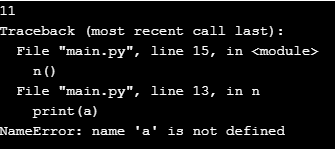##### Global variables in Python:

The variables which are declared outside of the function are called global variables. Global variables can be accessed in all functions of that module.

###### Example: Global variables in Python (Demo29.py)
```a=11
b=12
def m():
print("a from function m(): ",a)
print("b from function m(): ",b)
def n():
print("a from function n(): ",a)
print("b from function n(): ",b)
m()
n()```
###### Output: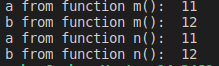##### GLOBAL KEYWORD IN PYTHON

The keyword global can be used for the following 2 purposes:

1. To declare a global variable inside a function
2. To make global variables available to the function.
##### When can we choose a global keyword?

There might be some scenarios, where the global variable names and local variable names are the same. In such cases, within the function, by default, the local variables are only referred and the global variables are ignored. If we want our function to refer to the global variable rather than the local variable, we can use the global keyword before the variable in the function as shown in demo31.py

###### Example: Global and Local variables having the same name in Python (Demo30.py)
```a=1
def m1():
a=2
print("a value from m1() function: ", a)
def m2():
print("a value from m2() function:", a)
m1()
m2()```
###### Output: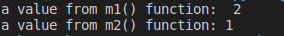Note: If a programmer wants to use the global variable inside a function, then need to use a global keyword before the variable in the beginning of the function body.

###### Example: global and local variables having the same name in Python (Demo31.py)
```a=1
def m1():
global a
a=2
print("a value from m1() function: ", a)
def m2():
print("a value from m2() function:", a)
m1()
m2()```
###### Output: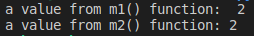If we use the global keyword inside the function, then the function is able to read-only global variables.

PROBLEM: This would make the local variable no more available.

##### globals() built-in function in python:

The problem of local variables not available, due to the use of global keywords can be overcome by using the Python built-in function called globals(). The globals() is a built-in function that returns a table of current global variables in the form of a dictionary. Using this function, we can refer to the global variable “a” as: global()[“a”].

###### Examle: Globals built-in function in python (Demo32.py)
```a=1
def m():
a=2
print(a)
print(globals()["a"])

m()```
###### Output: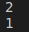### 1 thought on “Types of Variables in Python”

1.Arindam Kashyap

Can I give more than one global variable in the last function?
like this:

x=13
y=14
def variable_check_function_two():
x=12
print(“The value of Global variable X and Y is:”, globals()[“x””y”])
print(“The value of x and y is:”,x,y)

But, Its showing a TypeError! can you please give me the solution?